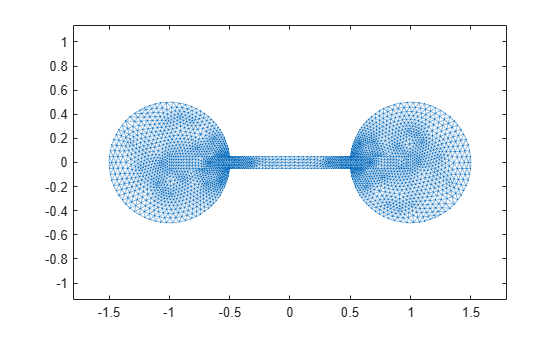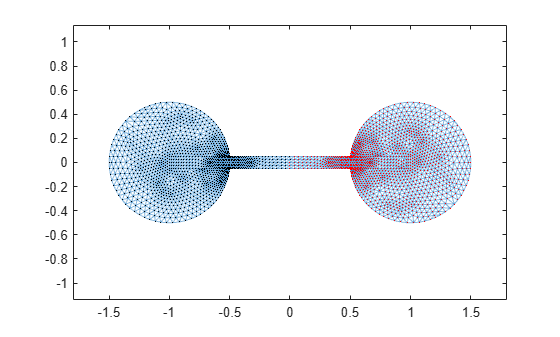# Partition Graph with Laplacian Matrix

This example shows how to use the Laplacian matrix of a graph to compute the Fiedler vector. The Fiedler vector can be used to partition the graph into two subgraphs.

Load the data set `barbellgraph.mat`, which contains the sparse adjacency matrix and node coordinates of a barbell graph.

`load barbellgraph.mat`

### Plot Graph

Plot the graph using the custom node coordinates `xy`.

```G = graph(A,'omitselfloops'); p = plot(G,'XData',xy(:,1),'YData',xy(:,2),'Marker','.'); axis equal```### Calculate Laplacian Matrix and Fiedler Vector

Calculate the Laplacian matrix of the graph. Then, calculate the two smallest magnitude eigenvalues and corresponding eigenvectors using `eigs`.

```L = laplacian(G); [V,D] = eigs(L,2,'smallestabs');```

The Fiedler vector is the eigenvector corresponding to the second smallest eigenvalue of the graph. The smallest eigenvalue is zero, indicating that the graph has one connected component. In this case, the second column in `V` corresponds to the second smallest eigenvalue `D(2,2)`.

`D`
```D = 2×2 10-3 × 0.0000 0 0 0.2873 ```
`w = V(:,2);`

Finding the Fiedler vector using `eigs` is scalable to larger graphs, since only a subset of the eigenvalues and eigenvectors are computed, but for smaller graphs it is equally feasible to convert the Laplacian matrix to full storage and use `eig(full(L))`.

### Partition Graph

Partition the graph into two subgraphs using the Fiedler vector `w`. A node is assigned to subgraph A if it has a positive value in `w`. Otherwise, the node is assigned to subgraph B. This practice is called a sign cut or zero threshold cut. The sign cut minimizes the weight of the cut, subject to the upper and lower bounds on the weight of any nontrivial cut of the graph.

Partition the graph using the sign cut. Highlight the subgraph of nodes with `w>=0` in red, and the nodes with `w<0` in black.

```highlight(p,find(w>=0),'NodeColor','r') % subgraph A highlight(p,find(w<0),'NodeColor','k') % subgraph B```For the bar bell graph, this partition bisects the graph nicely into two equal sets of nodes. However, the sign cut does not always produce a balanced cut.

It is always possible to bisect a graph by calculating the median of `w` and using it as a threshold value. This partition is called the median cut, and it guarantees an equal number of nodes in each subgraph.

You can use the median cut by first shifting the values in `w` by the median:

` w_med = w - median(w);`

Then, partition the graph by sign in `w_med`. For the bar bell graph, the median of `w` is close to zero, so the two cuts produce similar bisections.